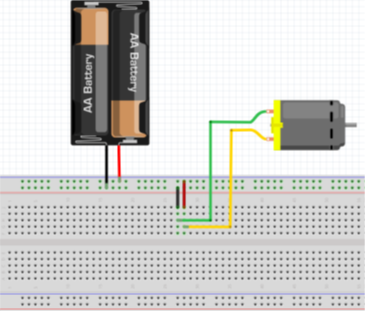## Powering a Motor

In the below circuit diagram, we are powering a brushless motor with a 20V battery. The resistance across the motor when running without a load is 50 ohms.
1. How much current, in mA, will flow through the motor?
2. What is the power, in watts, dissipated by the motor?
3. If the motor ran for 15 minutes under the above conditions, what is the minimum battery capacity in mAh?Hint
Ohm's law:
$$V=IR$$$where $$V$$ is the voltage, $$I$$ is the current, and $$R$$ is the resistance. Hint 2 Power absorbed by a resistive element: $$P=VI$$$
where $$P$$ is power, $$V$$ is the voltage, and $$I$$ is the current.
To solve for the current, use Ohm's law:
$$V=IR$$$where $$V$$ is the voltage, $$I$$ is the current, and $$R$$ is the resistance. $$I=\frac{V}{R}=\frac{20V}{50\Omega}=0.4A=400mA$$$
Power absorbed by a resistive element:
$$P=VI$$$where $$P$$ is power, $$V$$ is the voltage, and $$I$$ is the current. Solving for power dissipated by the motor: $$P=20V (0.4A)=8W$$$
To solve for capacity:
$$400mA \times 15min \times \frac{1h}{60min}=100mAh$$\$
1. 400 mA
2. 8 W
3. 100 mAh